# artan.filter package¶

## artan.filter.filter_params module¶

class artan.filter.filter_params.HasCalculateLoglikelihood[source]

Bases: pyspark.ml.param.Params

Mixin for param for enabling loglikelihood calculation.

calculateLoglikelihood = Param(parent='undefined', name='calculateLoglikelihood', doc='When true, loglikelihood of residual will be calculated & added to output DataFrame. Default is false')
getCalculateLoglikelihood()[source]

Gets the value of loglikelihood calculation flag.

class artan.filter.filter_params.HasCalculateMahalanobis[source]

Bases: pyspark.ml.param.Params

Mixin for param for enabling mahalanobis calculation.

calculateMahalanobis = Param(parent='undefined', name='calculateMahalanobis', doc='When true, mahalanobis distance of residual will be calculated & added to output DataFrame.Default is false.')
getCalculateMahalanobis()[source]

Gets the value of mahalanobis calcuation flag.

class artan.filter.filter_params.HasCalculateSlidingLikelihood[source]

Bases: pyspark.ml.param.Params

Mixin param for enabling sliding likelihood calculation

calculateSlidingLikelihood = Param(parent='undefined', name='calculateSlidingLikelihood', doc='When true, sliding likelihood sum of residual will be calculated & added to output DataFrame. Default is false')
getCalculateSlidingLikelihood()[source]

Gets the value of sliding likelihood calculation flag

class artan.filter.filter_params.HasControlCol[source]

Bases: pyspark.ml.param.Params

Mixin for param for control column.

controlCol = Param(parent='undefined', name='controlCol', doc='Column name for specifying control vector')
getControlCol()[source]

Gets the value of control column or its default value.

class artan.filter.filter_params.HasControlFunctionCol[source]

Bases: pyspark.ml.param.Params

Mixin for param for control function column.

controlFunctionCol = Param(parent='undefined', name='controlFunctionCol', doc='Column name for specifying control matrix')
getControlFunctionCol()[source]

Gets the value of control function column or its default value.

class artan.filter.filter_params.HasFadingFactor[source]

Bases: pyspark.ml.param.Params

fadingFactor = Param(parent='undefined', name='fadingFactor', doc='Factor controlling the weight of older measurements. With larger factor, more weights willbe given to recent measurements. Typically, should be really close to 1')
getFadingFactor()[source]

Gets the value of fading factor or its default value.

class artan.filter.filter_params.HasInitialStateCovariance[source]

Bases: pyspark.ml.param.Params

Mixin for param initial covariance matrix.

getInitialStateCovariance()[source]

Gets the value of initial covariance matrix or its default value.

initialStateCovariance = Param(parent='undefined', name='initialStateCovariance', doc='Initial covariance matrix')
class artan.filter.filter_params.HasInitialStateCovarianceCol[source]

Bases: pyspark.ml.param.Params

Mixin for param for initial covariance column.

getInitialStateCovarianceCol()[source]

Gets the value of initial covariance column or its default value.

initialStateCovarianceCol = Param(parent='undefined', name='initialStateCovarianceCol', doc='Column name for initial state covariance matrix.')
class artan.filter.filter_params.HasInitialStateDistributionCol[source]

Bases: pyspark.ml.param.Params

Mixin for param for initial state distribution column.

getInitialStateDistributionCol()[source]

Gets the value of initial distribution column or its default value.

initialStateDistributionCol = Param(parent='undefined', name='initialStateDistributionCol', doc='Parameter for initial state distribution as struct col')
class artan.filter.filter_params.HasInitialStateMean[source]

Bases: pyspark.ml.param.Params

Mixin for initial state vector.

getInitialStateMean()[source]

Gets the value of initial state vector or its default value.

initialStateMean = Param(parent='undefined', name='initialStateMean', doc='Initial state vector')
class artan.filter.filter_params.HasInitialStateMeanCol[source]

Bases: pyspark.ml.param.Params

Mixin for param for initial state column.

getInitialStateMeanCol()[source]

Gets the value of initial state column or its default value.

initialStateMeanCol = Param(parent='undefined', name='initialStateMeanCol', doc='Column name for initial state vector.')
class artan.filter.filter_params.HasMeasurementCol[source]

Bases: pyspark.ml.param.Params

Mixin for param for measurement column.

getMeasurementCol()[source]

Gets the value of measurement column or its default value.

measurementCol = Param(parent='undefined', name='measurementCol', doc='Column name for measurement vector. Missing measurements are allowed with nulls in the data')
class artan.filter.filter_params.HasMeasurementModel[source]

Bases: pyspark.ml.param.Params

Mixin for param measurement model matrix.

getMeasurementModel()[source]

Gets the value of measurement model matrix or its default value.

measurementModel = Param(parent='undefined', name='measurementModel', doc='Measurement matrix, when multiplied with the state it should give the measurement vector')
class artan.filter.filter_params.HasMeasurementModelCol[source]

Bases: pyspark.ml.param.Params

Mixin for param for measurement model column.

getMeasurementModelCol()[source]

Gets the value of measurement model column or its default value.

measurementModelCol = Param(parent='undefined', name='measurementModelCol', doc='Column name for specifying measurement model from input DataFrame rather thana constant measurement model for all filters')
class artan.filter.filter_params.HasMeasurementNoise[source]

Bases: pyspark.ml.param.Params

Mixin for param measurement noise matrix.

getMeasurementNoise()[source]

Gets the value of measurement noise matrix or its default value.

measurementNoise = Param(parent='undefined', name='measurementNoise', doc='Measurement noise matrix')
class artan.filter.filter_params.HasMeasurementNoiseCol[source]

Bases: pyspark.ml.param.Params

Mixin for param for measurement noise column.

getMeasurementNoiseCol()[source]

Gets the value of measurement noise column or its default value.

measurementNoiseCol = Param(parent='undefined', name='measurementNoiseCol', doc='Column name for specifying measurement noise from input DataFrame rather thana constant measurement noise for all filters')
class artan.filter.filter_params.HasMultiStepPredict[source]

Bases: pyspark.ml.param.Params

Mixin for multi step predict

getMultiStepPredict()[source]

Gets the value of multi step predict

multiStepPredict = Param(parent='undefined', name='multiStepPredict', doc='Number of predict steps after a predict&update cycle')
class artan.filter.filter_params.HasMultipleModelAdaptiveEstimationEnabled[source]

Bases: pyspark.ml.param.Params

getMultipleModelAdaptiveEstimationEnabled()[source]

Gets the value of MMAE output mode flag

multipleModelAdaptiveEstimationEnabled = Param(parent='undefined', name='multipleModelAdaptiveEstimationEnabled', doc='Flag for enabling Multiple Model Adaptive Estimation (MMAE) output mode. When enabled,MMAE mode outputs a single state estimate from the output of all kalman states of the transformer.States are weighted based on their sliding likelihood')
class artan.filter.filter_params.HasMultipleModelMeasurementWindowDuration[source]

Bases: pyspark.ml.param.Params

getMultipleModelMeasurementWindowDuration()[source]

Gets the value of mmae measureent window duration

multipleModelMeasurementWindowDuration = Param(parent='undefined', name='multipleModelMeasurementWindowDuration', doc='Window duration for grouping measurements in same window for MMAE filter aggregation')
class artan.filter.filter_params.HasOutputSystemMatrices[source]

Bases: pyspark.ml.param.Params

Mixin for param for enabling the output of system matrices along with the state.

getOutputSystemMatrices()[source]

Gets the value of loglikelihood calculation flag.

outputSystemMatrices = Param(parent='undefined', name='outputSystemMatrices', doc='When true, the system matrices will be added to output DataFrame. Default is false')
class artan.filter.filter_params.HasProcessModel[source]

Bases: pyspark.ml.param.Params

Mixin for param process model matrix.

getProcessModel()[source]

Gets the value of process model matrix or its default value.

processModel = Param(parent='undefined', name='processModel', doc='Process model matrix, transitions the state to the next state when applied')
class artan.filter.filter_params.HasProcessModelCol[source]

Bases: pyspark.ml.param.Params

Mixin for param for process model column.

getProcessModelCol()[source]

Gets the value of process model column or its default value.

processModelCol = Param(parent='undefined', name='processModelCol', doc='Column name for specifying process model from input DataFrame rather thana constant measurement model for all filters')
class artan.filter.filter_params.HasProcessNoise[source]

Bases: pyspark.ml.param.Params

Mixin for param process noise matrix.

getProcessNoise()[source]

Gets the value of process noise matrix or its default value.

processNoise = Param(parent='undefined', name='processNoise', doc='Process noise matrix')
class artan.filter.filter_params.HasProcessNoiseCol[source]

Bases: pyspark.ml.param.Params

Mixin for param for process noise column.

getProcessNoiseCol()[source]

Gets the value of process noise column or its default value.

processNoiseCol = Param(parent='undefined', name='processNoiseCol', doc='Column name for specifying process noise from input DataFrame rather thana constant measurement noise for all filters')
class artan.filter.filter_params.HasSlidingLikelihoodWindow[source]

Bases: pyspark.ml.param.Params

Mixin param for sliding likelihood window duration

getSlidingLikelihoodWindow()[source]

Gets the value of sliding likelihood window

slidingLikelihoodWindow = Param(parent='undefined', name='slidingLikelihoodWindow', doc='Number of consecutive measurements to include in the total likelihood calculation')
class artan.filter.filter_params.KalmanFilterParams[source]

Mixin for kalman filter parameters

setCalculateLogLikelihood()[source]

Optionally calculate loglikelihood of each measurement & add it to output dataframe. Loglikelihood is calculated from residual vector & residual covariance matrix.

Not enabled by default.

Returns: KalmanFilter
setCalculateMahalanobis()[source]

Optionally calculate mahalanobis distance metric of each measurement & add it to output dataframe. Mahalanobis distance is calculated from residual vector & residual covariance matrix.

Not enabled by default.

Returns: KalmanFilter
setCalculateSlidingLikelihood()[source]

Optionally calculate a sliding likelihood across consecutive measurements

Default is false

Returns: KalmanFilter
setControlCol(value)[source]

Set the column for input control vectors.

Control vectors should have compatible size with control function (controlVectorSize). The product of control matrix & vector should produce a vector with stateSize. null values are allowed, which will result in state transition without control input.

Parameters: value – String KalmanFilter
setControlFunctionCol(value)[source]

Set the column for input control matrices.

Control matrices should have dimensions (stateSize, controlVectorSize). null values are allowed, which will result in state transition without control input

Parameters: value – String KalmanFilter
setFadingFactor(value)[source]

Fading factor for giving more weights to more recent measurements. If needed, it should be greater than one. Typically set around 1.01 ~ 1.05. Default is 1.0, which will result in equally weighted measurements.

Parameters: value – Float >= 1.0 KalmanFilter
setInitialStateCovariance(value)[source]

Set the initial covariance matrix with dimensions (stateSize, stateSize)

It will be applied to all states. If the state timeouts and starts receiving measurements after timeout, it will again start from this initial covariance vector. Default is identity matrix. :param value: pyspark.ml.linalg.Matrix with dimensions (stateSize, stateSize) :return: KalmanFilter

setInitialStateCovarianceCol(value)[source]

Set the column corresponding to initial covariance matrix. Overrides setInitialCovariance parameter.

Parameters: value – String KalmanFilter
setInitialStateDistributionCol(value)[source]

Set the column corresponding to initial state distribution struct. The struct should have ‘mean’ field with a VectorType and ‘covariance’ field with a MatrixType. Overrides setInitialStateMeanCol and setInitialCovarianceCol parameters.

Parameters: value – String KalmanFilter
setInitialStateMean(value)[source]

Set the initial state vector with size (stateSize).

It will be applied to all states. If the state timeouts and starts receiving measurements after timeout, it will again start from this initial state vector. Default is zero.

Note that if this parameter is set through here, it will result in same initial state for all filters. For different initial states across filters, set the dataframe column for corresponding to initial state with setInitialStateCol.

Parameters: value – pyspark.ml.linalg.Vector with size (stateSize) KalmanFilter
setInitialStateMeanCol(value)[source]

Set the column corresponding to initial state vector. Overrides setInitialState setting.

Parameters: value – String KalmanFilter
setMeasurementCol(value)[source]

Set the column corresponding to measurements.

The vectors in the column should be of size (measurementSize). null values are allowed, which will result in only state prediction step.

Parameters: value – pyspark.ml.linalg.Vector with size measurementSize KalmanFilter
setMeasurementModel(value)[source]

Set default value for measurement model matrix with dimensions (stateSize, measurementSize) which maps states to measurement.

Note that if this parameter is set through here, it will result in same measurement model for all filters & measurements. For different measurement models across filters or measurements, set a dataframe column for measurement model from setMeasurementModelCol.

Default value maps the first state value to measurements.

Parameters: value – pyspark.ml.linalg.Matrix with dimensions (stateSize, measurementSize) KalmanFilter
setMeasurementModelCol(value)[source]

Set the column for input measurement model matrices

Measurement model matrices should have dimensions (stateSize, measurementSize)

Parameters: value – String KalmanFilter
setMeasurementNoise(value)[source]

Set default value for measurement noise matrix with dimensions (measurementSize, measurementSize).

Note that if this parameter is set through here, it will result in same measurement noise for all filters & measurements. For different measurement noise values across filters or measurements, set a dataframe column for measurement noise from setMeasurementNoiseCol.

Default is identity matrix.

Parameters: value – pyspark.ml.linalg.Matrix with dimensions (measurementSize, measurementSize) KalmanFilter
setMeasurementNoiseCol(value)[source]

Set the column for input measurement noise matrices.

Measurement noise matrices should have dimensions (measurementSize, measurementSize)

Parameters: value – String KalmanFilter
setMultiStepPredict(value)[source]

Sets the param for running multiple prediction steps after measurement updates in Kalman filter. By default, a single prediction step is followed by a single measurement update step. If this parameter is set to a number n>0, then n-predict steps will be undertaken after measurement update steps.

Default is 0 :param value: Integer :return: KalmanFilter

setOutputSystemMatrices()[source]

Optionally add system matrices to output dataframe returned by the transformer.

Default is false

Returns: KalmanFilter
setProcessModel(value)[source]

Set default value for process model matrix with dimensions (stateSize, stateSize) which governs state transition.

Note that if this parameter is set through here, it will result in same process model for all filters & measurements. For different process models across filters or measurements, set a dataframe column for process model from setProcessModelCol.

Default is identity matrix.

Parameters: value – pyspark.ml.linalg.Matrix with dimensions (stateSize, stateSize) KalmanFilter
setProcessModelCol(value)[source]

Set the column for input process model matrices.

Process model matrices should have dimensions (stateSize, stateSize)

Parameters: value – String KalmanFilter
setProcessNoise(value)[source]

Set default value for process noise matrix with dimensions (stateSize, stateSize).

Note that if this parameter is set through here, it will result in same process noise for all filters & measurements. For different process noise values across filters or measurements, set a dataframe column for process noise from setProcessNoiseCol.

Default is identity matrix.

Parameters: value – pyspark.ml.linalg.Matrix with dimensions (stateSize, StateSize) KalmanFilter
setProcessNoiseCol(value)[source]

Set the column for input process noise matrices.

Process noise matrices should have dimensions (stateSize, stateSize)

Parameters: value – String KalmanFilter
setSlidingLikelihoodWindow(value)[source]

Set the param for number of consecutive measurements to include in the total likelihood calculation

Default is 1

Parameters: value – Integer KalmanFilter

## artan.filter.least_mean_squares_filter module¶

class artan.filter.least_mean_squares_filter.LeastMeanSquaresFilter(featuresSize)[source]

Bases: artan.state.stateful_transformer.StatefulTransformer, artan.filter.filter_params.HasInitialStateMean, artan.filter.filter_params.HasInitialStateMeanCol, artan.filter.least_mean_squares_filter._HasLearningRate, artan.filter.least_mean_squares_filter._HasRegularizationConstant, pyspark.ml.param.shared.HasLabelCol, pyspark.ml.param.shared.HasFeaturesCol

Normalized Least Mean Squares filter, implemented with a stateful spark Transformer for running parallel filters /w spark dataframes. Transforms an input dataframe of observations to a dataframe of model parameters using stateful spark transformations, which can be used in both streaming and batch applications.

Belonging to stochastic gradient descent type of methods, LMS minimizes SSE on each measurement based on the expectation of steepest descending gradient.

Let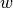denote the model parameter vector,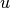denote the features vector, and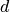the label corresponding to. Normalized LMS computesat step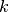recursively by: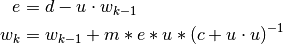Where -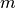: Learning rate -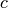: Regularization constant

setFeaturesCol(value)[source]

Set features column. Default is “features”

Parameters: value – String RecursiveLeastSquaresFilter
setInitialEstimate(value)[source]

Set initial estimate for model parameters. Default is zero vector.

Note that if this parameter is set through here, it will result in same initial estimate for all filters. For different initial estimates across filters, set the dataframe column for corresponding to initial estimate with setInitialEstimateCol.

Parameters: value – pyspark.ml.linalg.Vector with size (featuresSize) RecursiveLeastSquaresFilter
setInitialEstimateCol(value)[source]

Sets the column corresponding to initial estimates :param value: String :return: RecursiveLeastSquaresFilter

setLabelCol(value)[source]

Set label column. Default is “label”

Parameters: value – String RecursiveLeastSquaresFilter
setLearningRate(value)[source]

Set learning rate controlling the speed of convergence. Without noise, 1.0 is optimal.

Default is 1.0

Parameters: value – Float RecursiveLeastSquaresFilter
setRegularizationConstant(value)[source]

Set constant for regularization controlling stability. Larger values increase stability but degrade convergence performance. Generally set to a small constant.

Default is 1.0

Parameters: value – Float RecursiveLeastSquaresFilter

## artan.filter.linear_kalman_filter module¶

class artan.filter.linear_kalman_filter.LinearKalmanFilter(stateSize, measurementSize)[source]

Linear Kalman Filter, implemented with a stateful spark Transformer for running parallel filters /w spark dataframes. Transforms an input dataframe of noisy measurements to dataframe of state estimates using stateful spark transformations, which can be used in both streaming and batch applications.

Assuming a state vector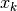with size stateSize, and measurements vector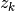with size measurementSize, below parameters can be specified.

•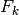, process model, matrix with dimensions stateSize x stateSize
•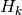, measurement model, matrix with dimensions stateSize x measurementSize
•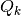, process noise covariance, matrix with dimensions stateSize x stateSize
•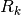, measurement noise covariance, matrix with dimensions measurementSize x measurementSize
•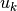, optional control vector, vector with size controlSize
•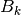, optional control model, matrix with dimensions stateSize x controlSize

Linear Kalman Filter will predict & estimate the state according to following state and measurement equations.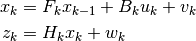Where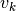and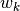are noise vectors drawn from zero mean,andcovariance distributions.

The default values of system matrices will not give you a functioning filter, but they will be initialized with reasonable values given the state and measurement sizes. All of the inputs to the filter can be specified with a dataframe column which will allow you to have different value across measurements/filters, or you can specify a constant value across all measurements/filters.

setEnableMultipleModelAdaptiveEstimation()[source]

Enable MMAE output mode

Returns: LinearKalmanFilter
setMultipleModelMeasurementWindowDuration(value)[source]

Optionally set the window duration for grouping measurements in same window for MMAE filter aggregation. Could be used for limiting the state on streaming if event time column is set.

Parameters: value – String LinearKalmanFilter

## artan.filter.recursive_least_squares_filter module¶

class artan.filter.recursive_least_squares_filter.RecursiveLeastSquaresFilter(featuresSize)[source]

Bases: artan.state.stateful_transformer.StatefulTransformer, artan.filter.filter_params.HasInitialStateMean, artan.filter.filter_params.HasInitialStateMeanCol, artan.filter.recursive_least_squares_filter._HasForgettingFactor, artan.filter.recursive_least_squares_filter._HasRegularizationMatrix, artan.filter.recursive_least_squares_filter._HasRegularizationMatrixCol, pyspark.ml.param.shared.HasLabelCol, pyspark.ml.param.shared.HasFeaturesCol, artan.filter.filter_params.HasInitialStateDistributionCol

Recursive formulation of least squares with exponential weighting & regularization, implemented with a stateful spark Transformer for running parallel filters /w spark dataframes. Transforms an input dataframe of observations to a dataframe of model parameters using stateful spark transformations, which can be used in both streaming and batch applications.

Letdenote the model parameters and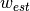denote our prior belief. RLS minimizes following regularization and weighted SSE terms;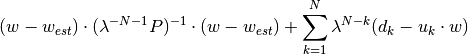Where:

•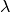: forgetting factor, or exponential weighting factor. Between 0 and 1.
•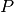: regularization matrix. Smaller values increseas the weight of regularization term, whereas larger
values increase the weight of weighted SSE term.
•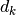,: label and features vector at time step k.
setFeaturesCol(value)[source]

Set features column. Default is “features”

Parameters: value – String RecursiveLeastSquaresFilter
setForgettingFactor(value)[source]

Set forgetting factor, exponentially weights the measurements based on its sequence.

Default value of 1.0 weights all measurements equally. With smaller values, recent measurements will have more weight. Generally set around 0.95 ~ 0.99

Parameters: value – Float >= 1.0 RecursiveLeastSquaresFilter
setInitialEstimate(value)[source]

Set initial estimate for model parameters. Default is zero vector.

Note that if this parameter is set through here, it will result in same initial estimate for all filters. For different initial estimates across filters, set the dataframe column for corresponding to initial estimate with setInitialEstimateCol.

Parameters: value – pyspark.ml.linalg.Vector with size (featuresSize) RecursiveLeastSquaresFilter
setInitialEstimateCol(value)[source]

Sets the column corresponding to initial estimates :param value: String :return: RecursiveLeastSquaresFilter

setInitialStateDistributionCol(value)[source]

Set the column corresponding to initial state distribution struct. The struct should have ‘mean’ field with a VectorType and ‘covariance’ field with a MatrixType. Overrides setInitialEstimateCol and setRegularizationMatrixCol parameters.

Parameters: value – String RecursiveLeastSquaresFilter
setLabelCol(value)[source]

Set label column. Default is “label”

Parameters: value – String RecursiveLeastSquaresFilter
setRegularizationMatrix(value)[source]

Set regularization matrix governing the influence of the initial estimate (prior). Larger values will remove regularization effect, making the filter behave like OLS.

Default is 10E5 * I

Note that if this parameter is set through here, it will result in same regularization for all filters. For different regularizations across filters, set the dataframe column for corresponding to regularization with setRegularizationMatrixCol.

Parameters: value – pyspark.ml.linalg.Matrix with size (featuresSize, featuresSize) RecursiveLeastSquaresFilter
setRegularizationMatrixCol(value)[source]

Sets the column corresponding to regularization matrix :param value: String :return: RecursiveLeastSquaresFilter

setRegularizationMatrixFactor(value)[source]

Sets the regularization matrix with a float factor, which results in setting the regularization matrix as factor * identity

Parameters: value – Float RecursiveLeastSquaresFilter